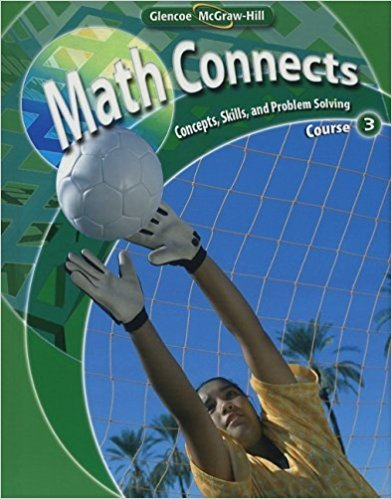×
×

# Solutions for Chapter 5: Math Connects: Concepts, Skills, and Problem Solving Course 3 0th Edition## Full solutions for Math Connects: Concepts, Skills, and Problem Solving Course 3 | 0th Edition

ISBN: 9780078740503Solutions for Chapter 5

Solutions for Chapter 5
4 5 0 342 Reviews
16
1
##### ISBN: 9780078740503

Chapter 5 includes 11 full step-by-step solutions. This textbook survival guide was created for the textbook: Math Connects: Concepts, Skills, and Problem Solving Course 3, edition: 0. This expansive textbook survival guide covers the following chapters and their solutions. Math Connects: Concepts, Skills, and Problem Solving Course 3 was written by and is associated to the ISBN: 9780078740503. Since 11 problems in chapter 5 have been answered, more than 1167 students have viewed full step-by-step solutions from this chapter.

Key Math Terms and definitions covered in this textbook
• Associative Law (AB)C = A(BC).

Parentheses can be removed to leave ABC.

• Big formula for n by n determinants.

Det(A) is a sum of n! terms. For each term: Multiply one entry from each row and column of A: rows in order 1, ... , nand column order given by a permutation P. Each of the n! P 's has a + or - sign.

• Characteristic equation det(A - AI) = O.

The n roots are the eigenvalues of A.

A sequence of steps (end of Chapter 9) to solve positive definite Ax = b by minimizing !x T Ax - x Tb over growing Krylov subspaces.

• Cyclic shift

S. Permutation with S21 = 1, S32 = 1, ... , finally SIn = 1. Its eigenvalues are the nth roots e2lrik/n of 1; eigenvectors are columns of the Fourier matrix F.

• Hilbert matrix hilb(n).

Entries HU = 1/(i + j -1) = Jd X i- 1 xj-1dx. Positive definite but extremely small Amin and large condition number: H is ill-conditioned.

• Inverse matrix A-I.

Square matrix with A-I A = I and AA-l = I. No inverse if det A = 0 and rank(A) < n and Ax = 0 for a nonzero vector x. The inverses of AB and AT are B-1 A-I and (A-I)T. Cofactor formula (A-l)ij = Cji! detA.

• Lucas numbers

Ln = 2,J, 3, 4, ... satisfy Ln = L n- l +Ln- 2 = A1 +A~, with AI, A2 = (1 ± -/5)/2 from the Fibonacci matrix U~]' Compare Lo = 2 with Fo = O.

• Minimal polynomial of A.

The lowest degree polynomial with meA) = zero matrix. This is peA) = det(A - AI) if no eigenvalues are repeated; always meA) divides peA).

• Nilpotent matrix N.

Some power of N is the zero matrix, N k = o. The only eigenvalue is A = 0 (repeated n times). Examples: triangular matrices with zero diagonal.

• Orthonormal vectors q 1 , ... , q n·

Dot products are q T q j = 0 if i =1= j and q T q i = 1. The matrix Q with these orthonormal columns has Q T Q = I. If m = n then Q T = Q -1 and q 1 ' ... , q n is an orthonormal basis for Rn : every v = L (v T q j )q j •

• Outer product uv T

= column times row = rank one matrix.

• Pascal matrix

Ps = pascal(n) = the symmetric matrix with binomial entries (i1~;2). Ps = PL Pu all contain Pascal's triangle with det = 1 (see Pascal in the index).

• Pivot columns of A.

Columns that contain pivots after row reduction. These are not combinations of earlier columns. The pivot columns are a basis for the column space.

• Pivot.

The diagonal entry (first nonzero) at the time when a row is used in elimination.

• Plane (or hyperplane) in Rn.

Vectors x with aT x = O. Plane is perpendicular to a =1= O.

• Symmetric matrix A.

The transpose is AT = A, and aU = a ji. A-I is also symmetric.

• Transpose matrix AT.

Entries AL = Ajj. AT is n by In, AT A is square, symmetric, positive semidefinite. The transposes of AB and A-I are BT AT and (AT)-I.

v + w = (VI + WI, ... , Vn + Wn ) = diagonal of parallelogram.

• Wavelets Wjk(t).

Stretch and shift the time axis to create Wjk(t) = woo(2j t - k).

×